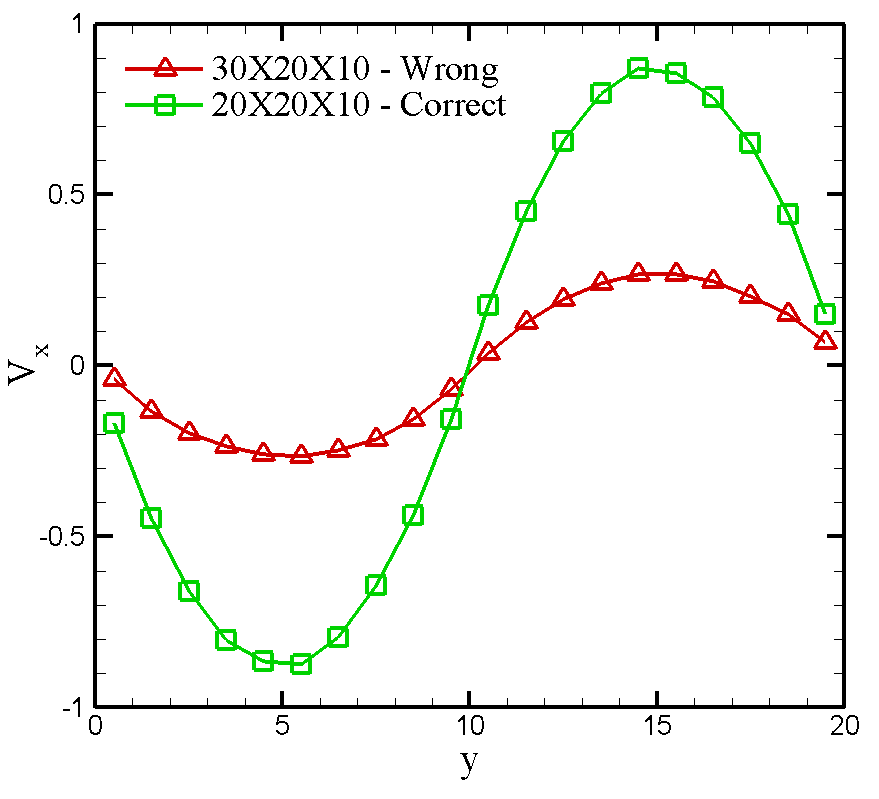# A Lammps bug for running periodic Poiseuille flow in Versions 2014-2016

I am reporting a bug in Lammps for running periodic Poiseuille flow.

Let x be the direction of applying force. When I use a computational box with Lx > Ly, the velocity profiles are wrong. However, if I use a box with Lx <= Ly, the simulation gives correct result.

For example, the following input script performs a periodic Poiseuille flow of a classic DPD fluid whose kinematic viscosity is 0.285. The simulation box is 30 X 20 X 10. Since Lx is larger than Ly, the simulation yields a wrong result with a maximum velocity of 0.276. However, if I use a box 20 X 20 X 10, the simulation gives correct velocity profile with the maximum velocity of 0.877.

I have tested the case on Lammps versions 2014-2016, all the versions have this bug. The bug makes Lammps give wrong results if Lx > Ly.

Below I paste the input script for showing the bug.

Best,
Zhen

I am reporting a bug in Lammps for running periodic Poiseuille flow.

Let x be the direction of applying force. When I use a computational box
with Lx > Ly, the velocity profiles are wrong. However, if I use a box with
Lx <= Ly, the simulation gives correct result.

For example, the following input script performs a periodic Poiseuille flow
of a classic DPD fluid whose kinematic viscosity is 0.285. The simulation
box is 30 X 20 X 10. Since Lx is larger than Ly, the simulation yields a
wrong result with a maximum velocity of 0.276. However, if I use a box 20 X
20 X 10, the simulation gives correct velocity profile with the maximum
velocity of 0.877.

I have tested the case on Lammps versions 2014-2016, all the versions have
this bug. The bug makes Lammps give wrong results if Lx > Ly.

Below I paste the input script for showing the bug.

unfortunately, your input script itself has a problem:
these two lines cannot be executed due to missing variable definitions.

variable ymid equal (\{yhi\}\+{ylo})/2
variable fx atom mass*\{gx\}\*\(\(y&gt;{ymid})-(y<${ymid})) axel. Hi Axel, Thanks for your reply. I deleted the definition of yhi and ylo, please replace the two line with following lines variable ymid equal 10.0 variable fx atom mass*{gx}*((y>{ymid})-(y<${ymid})

Value of gx was also deleted. Please add one following line before defining variable fx. Thanks.

variable gx equal 0.02

Value of gx was also deleted. Please add one following line before defining variable fx. Thanks.

variable gx equal 0.02

thanks. i finally was able to run those two input variants, but
comparison of the log files shows, that they are not meaningful to
compare.
you create the same number of atoms in both cases, so you have
significantly different densities and consequently different velocity
profiles.

so i don't see convincing evidence for a bug anywhere.

axel.

Hi, Axel,

The bug I mentioned refers to the exactly same system density \rho=3, so box 30X20X10 uses 18000 particles, 20X20X10 uses 12000 particles.

Here I am sending you the two input scripts that your can run them directly. And you can diff the two files to see their difference: only 30 is changed to 20.

The following shows some screen outputs:dpd_30X20X10_wrong.in (2.98 KB)

dpd_20X20X10_correct.in (2.98 KB)